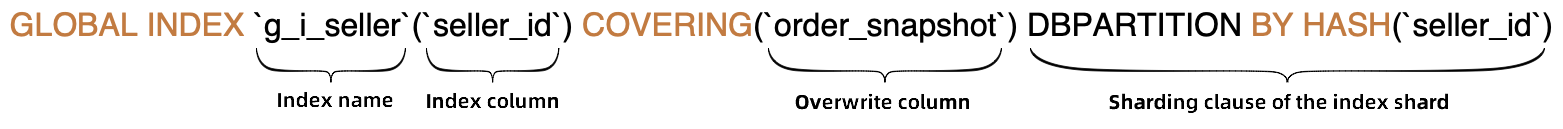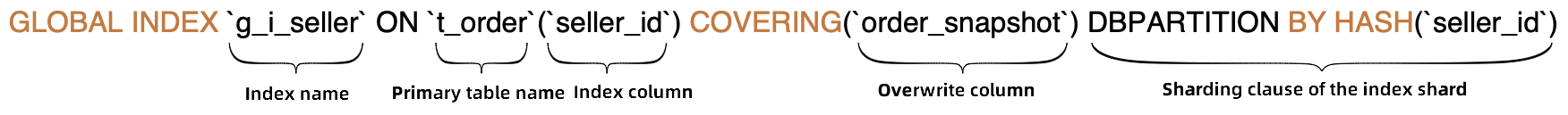DRDS supports global secondary indexes (GSIs). This topic describes how to create GSIs and use the GSI feature.

## Prerequisites

The MySQL version must be 5.7 or later, and the minor version of the kernel must be 5.4.1 or later.

## Create a GSI

DRDS has extended MySQL data definition language (DDL) syntax by adding syntax that is used to define GSIs. The syntax usage is the same as the usage of syntax that is used to create indexes on MySQL.

• Define a GSI when you create a table• Add a GSI after you create a tableNote
• Index name: used as the name of an index table to create the index table.
• Index column: the shard key of the index table, that is, all the columns that are used in the sharding clause of the index.
• Covering column: other columns in the index table. By default, the primary key and all the shard keys of the primary table are included.
• Sharding clause of the index: the sharding algorithm of the index table. Its syntax is the same as the syntax of the sharding clause in CREATE TABLE.

Examples

``````# Define a GSI when you create a table.
CREATE TABLE t_order (
`id` bigint(11) NOT NULL AUTO_INCREMENT,
`order_id` varchar(20) DEFAULT NULL,
`seller_id` varchar(20) DEFAULT NULL,
`order_snapshot` longtext DEFAULT NULL,
`order_detail` longtext DEFAULT NULL,
PRIMARY KEY (`id`),
GLOBAL INDEX `g_i_seller`(`seller_id`) COVERING (`id`, `order_id`, `buyer_id`, `order_snapshot`) dbpartition by hash(`seller_id`)
) ENGINE=InnoDB DEFAULT CHARSET=utf8 dbpartition by hash(`order_id`);
COVERING(`seller_id`, `order_snapshot`)

## Use a GSI

After you create a GSI, you can enable a query to use an index table by using the following methods:

• Use HINT to specify indexes

You can choose one of the following two HINT statements to specify and use the index for the query:

• Statement:
``FORCE INDEX({index_name})``
Example:
``````SELECT a.*, b.order_id
FROM t_seller a
JOIN t_order b FORCE INDEX(g_i_seller) ON a.seller_id = b.seller_id
WHERE a.seller_nick="abc";``````
• Syntax
``/*+TDDL:INDEX({table_name/table_alias}, {index_name})*/``
Example:
``/*+TDDL:index(a, g_i_buyer)*/ SELECT * FROM t_order a WHERE a.buyer_id = 123``
Note If a query requires the columns that are not contained in an index, you can query the index table first to retrieve all the recorded primary keys and the shard keys of the primary table. Then, query the primary table to retrieve the values of the missing columns. For more information, see INDEX HINT.
• Directly query an index table

If the index table contains all the columns that are required for a query, you can directly query the index table to retrieve the result.

• Select an index

For a query on the primary table that has a GSI, DRDS automatically selects the index table that the optimizer considers to have the minimum cost. Only covering indexes can be selected.

In the following SQL query, the primary table is `t_order`. The `seller_id` equality filter is contained, and the involved columns such as `id`, `order_snapshot`, and `seller_id` are covered by the GSI `g_i_seller`. The use of the `g_i_seller` covering index eliminates the need to query the primary table and significantly reduces the number of scans on the table shardings. `seller_id` is the shard key of `g_i_seller`. The result of EXPLAIN shows that the DRDS optimizer selects `g_i_seller`.

``````EXPLAIN SELECT t_order.id,t_order.order_snapshot FROM t_order WHERE t_order.seller_id = 's1';
IndexScan(tables="g_i_seller_sfL1_2", sql="SELECT `id`, `order_snapshot` FROM `g_i_seller` AS `g_i_seller` WHERE (`seller_id` = ?)")          ``````
• IGNORE INDEX and USE INDEX

You can enable the optimizer to use or not to use some indexes by using the following HINT:

• Statement:
``IGNORE INDEX({index_name},...)``

Example:

``SELECT t_order.id,t_order.order_snapshot FROM t_order IGNORE INDEX(g_i_seller) WHERE t_order.seller_id = 's1';``
• Statement:
``USE INDEX({index_name},...)``
Example:
``SELECT t_order.id,t_order.order_snapshot FROM t_order USE INDEX(g_i_seller) WHERE t_order.seller_id = 's1';``